# AP Calculus BC Practice Test 21

### Test Information10 questions20 minutes

Calculator Disallowed

1.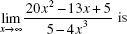2.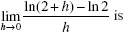3. If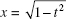and y = sin-1t, then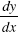equals

4. Use the following table, which shows the values of the differentiable functionsf and g.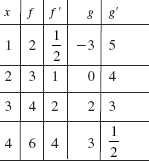The average rate of change of function f on [1,4] is

5. Use the following table, which shows the values of the differentiable functionsf and g.If h(x) = g(f (x)) then h (3) =

6.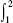(3x - 2)3 dx is equal to

7. If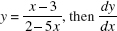equals

8. The maximum value of the function f (x) = xe -x is

9. Which equation has the slope field shown below?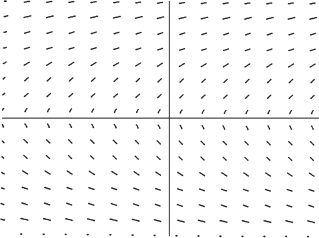10. The graph below shows the velocity of an object moving along a line, for 0 ≤ t ≤ 9.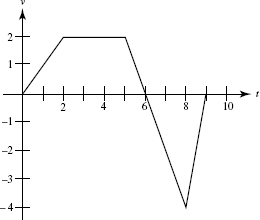At what time does the object attain its maximum acceleration?